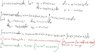# Integral of sin(x) sin(x+1) dx from 0 to 2pi.

• s3a

## Homework Statement

Integral of sin(x) sin(x+1) dx from 0 to 2pi.

## Homework Equations

Integration by parts: Integral u dv = uv – Integral v du

## The Attempt at a Solution

My work has been attached as MyWork.jpg. I, basically, get 0 * integral_I_started_with = something_else instead of nonzero_constant * integral_I_started_with = something_else and, given that I've done this problem a multitude of times and failed (if I remember correctly, always with the same issue) and, I was hoping someone here could point out my mistake.

Any input would be greatly appreciated!

#### Attachments

•MyWork.jpg
36.5 KB · Views: 3,837
s3a said:

## Homework Statement

Integral of sin(x) sin(x+1) dx from 0 to 2pi.

## Homework Equations

Integration by parts: Integral u dv = uv – Integral v du

## The Attempt at a Solution

My work has been attached as MyWork.jpg. I, basically, get 0 * integral_I_started_with = something_else instead of nonzero_constant * integral_I_started_with = something_else and, given that I've done this problem a multitude of times and failed (if I remember correctly, always with the same issue) and, I was hoping someone here could point out my mistake.

Any input would be greatly appreciated!

Try a different approach. There's a trig formula that will let you express sin(A)sin(B) as a sum of simple trig functions. Can you find it?

s3a said:

## Homework Statement

Integral of sin(x) sin(x+1) dx from 0 to 2pi.

## Homework Equations

Integration by parts: Integral u dv = uv – Integral v du

## The Attempt at a Solution

My work has been attached as MyWork.jpg. I, basically, get 0 * integral_I_started_with = something_else instead of nonzero_constant * integral_I_started_with = something_else and, given that I've done this problem a multitude of times and failed (if I remember correctly, always with the same issue) and, I was hoping someone here could point out my mistake.

Any input would be greatly appreciated!

That image is difficult to read and in any case you didn't put in the limits. But integration by parts isn't what you want to do anyway. Use the formula$$\sin a \sin b =\frac 1 2 (\cos(a-b)-\cos(a+b))$$

Your double integration by parts yielded
$$\int \sin(x)\sin(x+1)dx = -\sin(x+1)\cos(x) + \cos(x+1)\sin(x) + \int \sin(x)\sin(x+1)dx$$
(This is the line just before the line that is colored red.) Subtract the integral from both sides and you get
$$0 = -\sin(x+1)\cos(x) + \cos(x+1)\sin(x)$$
One may rightfully ask, what gives?

Look at the right hand side, ##-\sin(x+1)\cos(x) + \cos(x+1)\sin(x)##. This is ##\sin(x-(x+1))##, or ##\sin(1)##. So now we have the even more nonsensical ##\sin(1)=0##, which obviously is not true.

What happened is that you dropped a constant of integration. That supposedly nonsensical ##\sin(1)=0## is better stated as ##\sin(1) = c##. Instead of reaching a contradiction you've reached a tautology.

Bottom line: That's Just one of those things you need to be aware of when you do integration by parts.

Here's a hint that will help you solve this integral: Use the trick that x-x=0.
With this, cos(a) = cos(a+x-x) = cos((a+x)-x) = cos(a+x)cos(x) + sin(a+x)sin(x).
Now substitute a=1.

Thanks, guys!

An alternative approach.
Let
$$I=\int_{0}^{2\pi} \sin(x)\cdot\sin(x+1)dx$$

The integral is equivalent to
$$I=\int_{0}^{2\pi} \sin(2\pi-x)\cdot \sin(2\pi-(x-1))dx=\int_0^{2\pi} \sin(x)\cdot\sin(x-1)dx$$

Add the two expressions to get,
$$2I=\int_0^{2\pi} \sin(x)(\sin(x+1)+\sin(x-1))dx$$
Use ##\sin(A)+\sin(B)=2\sin((A+B)/2)\cos((A-B)/2)## to obtain,
$$2I=\int_0^{2\pi} \sin(x)(2\sin(x)\cos(1))dx \Rightarrow I=\cos(1)\int_0^{2\pi} \sin^2x dx$$

From the formula, ##\cos(2x)=1-2\sin^2x##, the integral can be easily evaluated.

I hope that helped.

Pranav-Arora said:
An alternative approach.
Let
$$I=\int_{0}^{2\pi} \sin(x)\cdot\sin(x+1)dx$$

The integral is equivalent to
$$I=\int_{0}^{2\pi} \sin(2\pi-x)\cdot \sin(2\pi-(x-1))dx=\int_0^{2\pi} \sin(x)\cdot\sin(x-1)dx$$

Add the two expressions to get,
$$2I=\int_0^{2\pi} \sin(x)(\sin(x+1)+\sin(x-1))dx$$
Use ##\sin(A)+\sin(B)=2\sin((A+B)/2)\cos((A-B)/2)## to obtain,
$$2I=\int_0^{2\pi} \sin(x)(2\sin(x)\cos(1))dx \Rightarrow I=\cos(1)\int_0^{2\pi} \sin^2x dx$$

From the formula, ##\cos(2x)=1-2\sin^2x##, the integral can be easily evaluated.

I hope that helped.

This problem could have been solved with much more ease, if you multiply the integral and divide by 2 and then isolate 1/2 out. Then apply formula 2sinAsinB=cos(A-B)-cos(A+B).

I know its too late to post here though.

Edit: Excellent hint given by LC Kurtz and Dick.